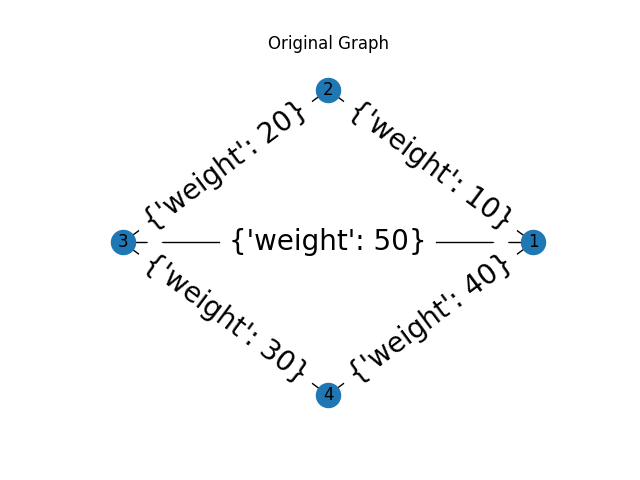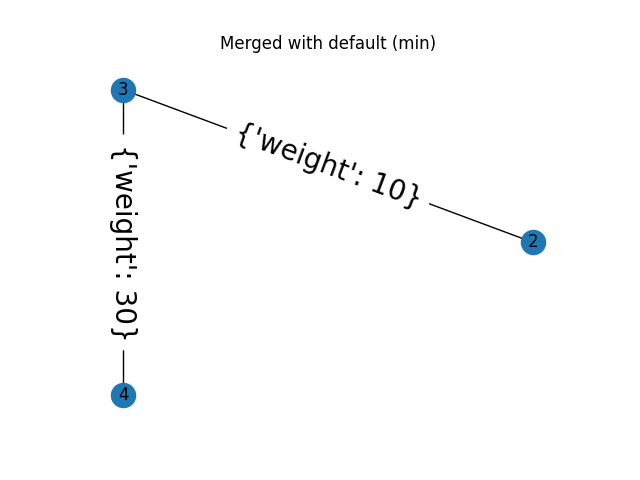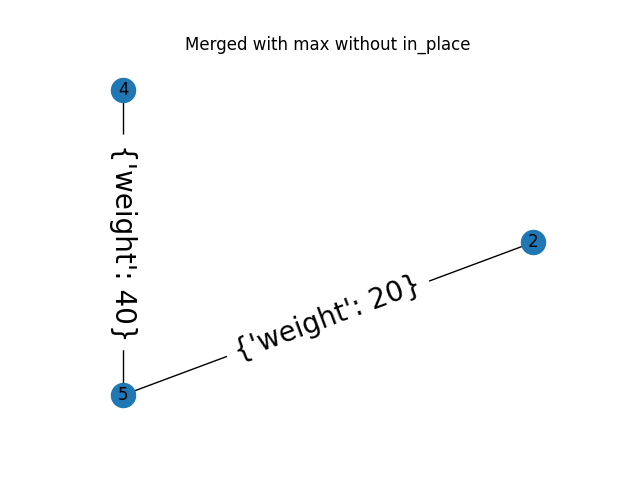This example demonstrates the use of the merge_nodes function of a Region Adjacency Graph (RAG). The RAG class represents a undirected weighted graph which inherits from `networkx.graph` class. When a new node is formed by merging two nodes, the edge weight of all the edges incident on the resulting node can be updated by a user defined function weight_func.

The default behaviour is to use the smaller edge weight in case of a conflict. The example below also shows how to use a custom function to select the larger weight instead.

•••```import skimage as ski
import networkx as nx
from matplotlib import pyplot as plt
import numpy as np

def max_edge(g, src, dst, n):
"""Callback to handle merging nodes by choosing maximum weight.

Returns a dictionary with `"weight"` set as either the weight between
(`src`, `n`) or (`dst`, `n`) in `g` or the maximum of the two when
both exist.

Parameters
----------
g : RAG
The graph under consideration.
src, dst : int
The vertices in `g` to be merged.
n : int
A neighbor of `src` or `dst` or both.

Returns
-------
data : dict
A dict with the "weight" attribute set the weight between
(`src`, `n`) or (`dst`, `n`) in `g` or the maximum of the two when
both exist.
"""

w1 = g[n].get(src, {'weight': -np.inf})['weight']
w2 = g[n].get(dst, {'weight': -np.inf})['weight']
return {'weight': max(w1, w2)}

def display(g, title):
"""Displays a graph with the given title."""
pos = nx.circular_layout(g)
plt.figure()
plt.title(title)
nx.draw(g, pos)
nx.draw_networkx_labels(g, pos)
nx.draw_networkx_edge_labels(g, pos, font_size=20)

g = ski.graph.RAG()

# Assigning dummy labels.
for n in g.nodes():
g.nodes[n]['labels'] = [n]

gc = g.copy()

display(g, "Original Graph")

g.merge_nodes(1, 3)
display(g, "Merged with default (min)")

gc.merge_nodes(1, 3, weight_func=max_edge, in_place=False)
display(gc, "Merged with max without in_place")

plt.show()
```

Total running time of the script: ( 0 minutes 0.126 seconds)

Gallery generated by Sphinx-Gallery